# The meaning of Bell's inequality

Published 2018/8/10

To be honest, Bell's inequality is an inequality that gives the upper limit of the probability that Rainy Woman and Sunny Man meet.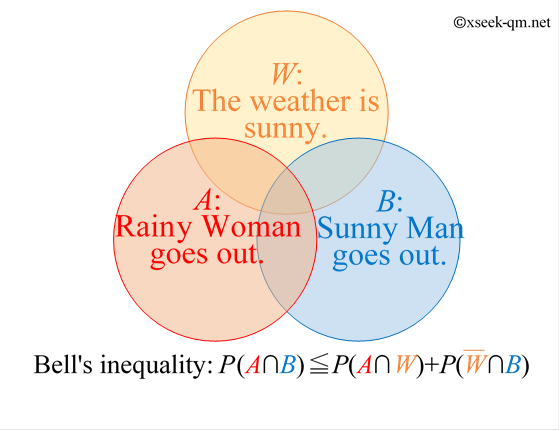Bell's inequality is often found in the introduction to quantum mechanics. However, I think the meaning of the inequality is very difficult to understand. So, in this article, I will try to explain the meaning of Bell's inequality easily.

## An explanation of Bell's inequality in everyday words

Bell's inequality is as follows.

Bell's inequality:
$$P(A \cap B) \leqq P(A \cap W)+P(\overline{W} \cap B)$$

(If it is different from Bell's inequalities you know, please read "Various Bell's Inequalities" below first)

The meaning of Bell's inequality is very unclear. One of the reasons is that the symbols $W$, $A$, $B$ are abstract expressions. So, I would like to explain Bell's inequality by replacing the symbols $W$, $A$, $B$ to the following everyday words.

• $W$: The weather is sunny.
• $A$: Alice goes out.
• $B$: Bob goes out.

$P(A \cap B)$ means the probability that Alice and Bob go out at the same time. $P(A \cap W)$ means the probability that the weather is sunny and Alice goes out. $P(\overline{W} \cap B)$ means the probability that weather is rainy and Bob goes out.

Bell's inequality can be expressed in everyday words as follows:

• The upper limit of the probability that Alice and Bob go out at the same time is the sum of the probability that the weather is sunny and Alice goes out, and the probability that Bob goes out and the weather is rainy.

The expression "probability that Alice and Bob go out at the same time" is not very interesting. So, I would like to think another expression "probability that Alice and Bob meet". "Probability that Alice and Bob meet" never exceeds "probability that Alice and Bob go out at the same time" no matter how high the probability. Therefore, the above statement can be said as follows.

• The upper limit of the probability that Alice and Bob meet is the sum of the probability that the weather is sunny and Alice goes out, and the probability that Bob goes out and the weather is rainy.

This means that "Even if the probability that Alice and Bob meet is high, it does not exceed the sum of the probability that the weather is sunny and Alice goes out and the probability that Bob goes out and the weather is rainy."

Now, let's consider the following case.

• When Alice goes out, it tends to be rainy for some reason. The women like Alice is called Rainy Woman in Japan.
• When Bob goes out, it tends to be sunny for some reason. THe man like Bob is called Sunny Man in Japan.

The Venn diagram looks like this:Then, Bell's inequality can be expressed by the following sentence.

• The upper limit of the probability that Rainy Woman and Sunny Man meet is the sum of the probability that the weather is sunny and the Rainy Woman goes out, and the probability that the weather is rainy and the Sunny Man goes out.

We can see the meaning of Bell's inequality in the following Venn diagram visually.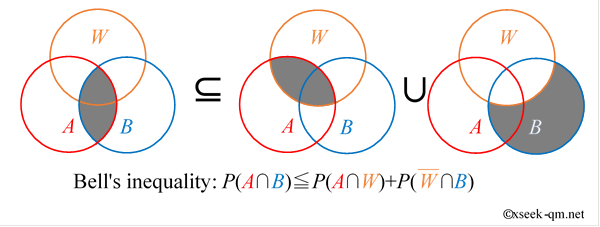## Question related to Bell's Inequality

Here, I would like to ask a question related to Bell's inequality.

### Problem

What is the upper limit of the probability that Alice and Bob meet when we have the following conditions of the question?

• The probability that the weather is sunny is 50%.
• The probability that Alice goes out is 50%.
• The probability that Bob goes out is 50%.
• The probability that the weather is rainy is 75% when Alice goes out because she is Rainy Woman.
• The probability that the weather is sunny is 75% when Bob goes out because he is Sunny Man.

## Answer to the question related to Bell's Inequality

The answer is 25%. I would like to explain how to solve the question.

As a precaution, I would like to reconfirm the answer that the question is expecting here. The answer to this question is not "probability." It is "upper limit of probability". Because it is the upper limit, you may consider the case that "Alice and Bob are easy to go out for some reason at the same time" or the case that "Alice and Bob have a tendency to meet for some reason". This question is asking the question, "How high can the probability that Alice and Bob meet as a maximum?"

We divide this question into the case of sunny day and the case of rainy day in order to calculate the conditional probability. First we consider the case of a sunny day.

### Case of sunny day

The probability that Alice goes out is 50%. It is expressed in the following formula.

$$P(A) = 50\%$$

The probability that the weather is rainy is 75% when Alice goes out. The conditional probability can be expressed by the following equation.

$$P(\overline{W}|A)=\frac{P(A \cap \overline{W})}{P(A)} = 75\%$$

On the other hand, the probability that the weather is sunny is 50%, so the probability that the weather is rainy is 50%.

$$P(\overline{W}) = 50\%$$

Therefore, the probability that Alice goes out on a rainy day is 75%.

$$P(A|\overline{W})=\frac{ P(\overline{W} \cap A)}{P(\overline{W})} = 75\%$$

From this, we can see that the probability that Alice goes out on a sunny day is 25%.

$$P(A|W)=\frac{ P(W \cap A)}{P(W)} = 25\%$$

Similarly, the probability that Bob goes out on a sunny day is 75%.

$$P(B|W)=\frac{ P(W \cap B)}{P(W)} = 75\%$$

For Alice and Bob to meet, they both need to be out. Therefore, on a sunny day, the upper limit of the probability that they both will meet is 25%.

### Case of rainy day

We consider like a sunny day.

The probability that Alice goes out on a sunny day is 75%.

$$P(A|\overline{W})=\frac{ P(\overline{W} \cap A)}{P(\overline{W})} = 75\%$$

On the other hand, the probability that Bob goes out on a rainy day is 25%.

$$P(B|\overline{W})=\frac{ P(\overline{W} \cap B)}{P(\overline{W})} = 25\%$$

For Alice and Bob to meet, they both need to be out. Therefore, on a rainy day, the upper limit of the probability that they both two will meet is 25%.

### If you combine the case of sunny day and the case of rainy day ...

If you combine the case of sunny day and the case of rainy day, you can see that the upper limit of the probability that they both meet is 25%.

$$P(W)\times P(A|W)+P(\overline{W})\times P(B|\overline{W})= 25\%$$

The above expression can be rewritten as follows.

$$P(A \cap W)+P(\overline{W} \cap B) = 25\%$$

So, the right hand side of Bell's inequality on this question is 25%.

Bell's inequality:
$$P(A \cap B) \leqq P(A \cap W)+P(\overline{W} \cap B) = 25\%$$

Therefore, the answer to "How much can you increase the probability that Alice and Bob meet?" is 25%. As long as the conditions of this question are kept, we can not get any further probability by any means. This conclusion is always correct as long as the weather can be divided into sunny and rainy days. But surprisingly, in quantum mechanics the left hand side of this inequality is 37.5%! I will explain it next.

## Mystery of EPR correlation

I would like to consider the following thought experiment based on quantum mechanics.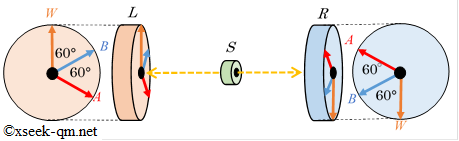The source $S$ emits one electron to the left observation device $L$ and the other electron to the right observation device $R$. The total of the angular momentum of two electrons is 0.

The observation devices can measure the spin of an electron in one direction. It is strange that the spin of any electron is always either $+1/2$ or $-1/2$. In the case of that the total of the angular momentum of two electrons is 0, the probability that the right observation device $R$ gets the spin $+1/2$ of one electron in the direction $W$ is 100%, when the left observation device $L$ gets the spin of the other electrons $+1/2$ in the direction $W$.

From here, the explanation becomes a little more difficult. The left observation device $L$ measure the spin of an electron in the direction $A$. On the other hand, the right observation devicce $R$ measure the spin of the other electron in the direction $B$. In the case of that the angle difference between the direction $A$ and direction $B$ is $\theta$, the probability that the right observation device $R$ gets the spin $+1/2$ of one electron in the direction $B$ can be calculated in the following formula in quantum mechanics, when the left observation device $L$ gets the spin $+1/2$ of the other electron in the direction $A$.

$$P(B|A) = \frac{ P(A \cap B)}{P(A)}= \cos^2 \left(\frac{\theta}{2} \right)$$

Here, $P(B|A)$ is the probability that the event $B$ occurs when the event $A$ occurs. And $P(A \cap B)$ is the probability that the event $A$ and the event $B$ occur at the same time. I would like to show some examples below.

• In the case of that the angle difference between the direction $A$ and direction $B$ is $0^\circ$, the probability that the right observation device $R$ gets the spin $+1/2$ of one electron in the direction $B$ is 100%, when the left observation device $L$ gets the spin $+1/2$ of the other electron in the direction $A$.
• In the case of that the angle difference between the direction $A$ and direction $B$ is $90^\circ$, the probability that the right observation device $R$ gets the spin $+1/2$ of one electron in the direction $B$ is 50%, when the left observation device $L$ gets the spin $+1/2$ of the other electron in the direction $A$.
• In the case of that the angle difference between the direction $A$ and direction $B$ is $180^\circ$, the probability that the right observation device $R$ gets the spin $+1/2$ of one electron in the direction $B$ is 0%, when the left observation device $L$ gets the spin $+1/2$ of the other electron in the direction $A$.

The angle difference between the direction $A$ and direction $B$ is $60^\circ$, so the probability that the right observation device $R$ gets the spin $+1/2$ of one electron in the direction $B$ is 75%, when the left observation device $L$ gets the spin $+1/2$ of the other electron in the direction $A$ as follows.

$$P(B|A) = \frac{ P(A \cap B)}{P(A)}=\cos^2 \left(\frac{60^\circ}{2} \right) = 75\%$$

The probability $P(A)$ is 1/2, so the probability $P(A \cap B)$ that both observation devices get the spin $+1/2$ of electrons at the same time is 37.5%.

$$P(A \cap B) = 37.5\%$$

Now I would like to consider another case. The left observation device $L$ measure the spin of an electron in the direction $A$. On the other hand, the right observation devicce $R$ measure the spin of the other electron in the direction $W$. The angle difference between the direction $A$ and direction $W$ is $120^\circ$, so the probability that the right observation device $R$ gets the spin $+1/2$ of one electron in the direction $B$ is 25%, when the left observation device $L$ gets the spin $+1/2$ of the other electron in the direction $A$ as follows.

$$P(W|A) = \frac{ P(A \cap W)}{P(A)}=\cos^2 \left(\frac{120^\circ}{2} \right) = 25\%$$

The probability $P(A)$ is 1/2, so the probability $P(A \cap W)$ that both observation devices get the spin $+1/2$ of electrons at the same time is 12.5%.

$$P(A \cap W) = 12.5\%$$

I would like to consider another case. The left observation device $L$ measure the spin of an electron in the opposite direction $W$. (From now, we use a new symbol $\overline{W}$ which means the opposite direction of $W$) On the other hand, the right observation devicce $R$ measure the spin of the other electron in the direction $B$. The angle difference between the direction $\overline{W}$ and direction $B$ is $120^\circ$, so the probability that the right observation device $R$ gets the spin $+1/2$ of one electron in the direction $B$ is 25%, when the left observation device $L$ gets the spin $+1/2$ of the other electron in the direction $\overline{W}$ as follows.

$$P(B|\overline{W}) = \frac{ P(\overline{W} \cap B)}{P(\overline{W})}=\cos^2 \left(\frac{120^\circ}{2} \right) = 25\%$$

$P(\overline{W})$ Is 1/2, so both results are $+1/2$ The probability of becoming is 12.5%.

$$P(\overline{W} \cap B) = 12.5\%$$

Here, Bell's inequality is:

Bell's inequality:
$$P(A \cap B) \leqq P(A \cap W)+P(\overline{W} \cap B).$$

The left hand side of the above inequality is 37.5%, but the sum of the right hand side is 25%, which breaks Bell's inequality. Therefore, a strange correlation that is impossible in nature exists between the observation device $L$ and the observation device $R$. This mysterious correlation is called EPR correlation and has been confirmed in actual experiments. (For example, it is confirmed in Aspect's experiment in 1982)

Where does the mystery of EPR correlation come from? I think it comes from that the measurement results $A$ and $B$ can not be divided by the results $W$ which is not measured. This is similar to the fact that the results can not be divided by the case that an electron go throuth the left slit and the case that an electron goes through the right slit in a double-slit experiment.

## Why Bell's inequality breaks?

Here, I would like to consider the reason why Bell's inequality breaks.

In the previous section, it is said that the mystery of EPR comes from that the measurement results $A$ and $B$ can not be divided by the results $W$ which is not measured. However, surprisingly we can separate the results $W$ which is not measured spatially. Before the explanation, I would like to explain how to measure the spin of electrons.

To measure the spin of electrons, use the following Stern-Gellerach device.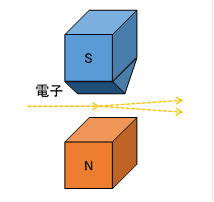An uneven magnetic field is generated between the pointed south pole and the flat north pole. The strength of the magnetic field becomes stronger as it approaches the south pole. The electron beam passing through the inhomogeneous magnetic field is split into two trajectories. The direction of the electron's spin can be found by the trajectory. Whatever direction the original spin is facing, it always splits into two trajectories relative to the direction of the magnet.

This Stern-Gellerach device is combined to make the following device.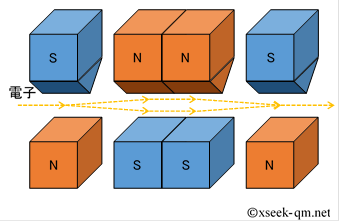In the above-mentioned device, the once branched electron beams merge again. For the sake of later, I will try to abbreviate the above device in the next figure.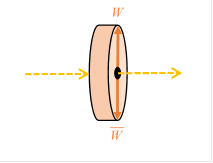We put the wall $C$ in the device.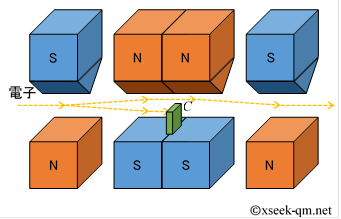The lower trajectory is blocked, so we can find which trajectory it passed. For the sake of simplicity, the above device is abbreviated in the following figure.The following thought experiment is performed using these devices.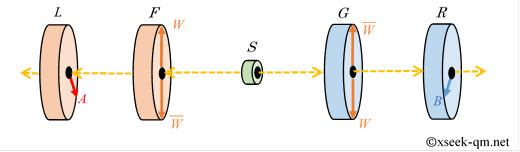Though electron beam is separated into the trajectory $W$ and the trajectory $\overline{W}$ and combined again by a device $F$ and a device $G$, EPR correlation still occurs between the device $L$ and the device $R$.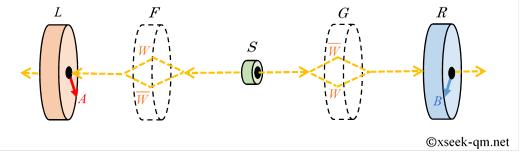This is a very strange result, because the electron beam could be divided into the case $W$ and the case $\overline{W}$ when the electron beam was separated into trajectory $W$ and trajectory $\overline{W}$. If the cases can be divided, Bell's inequality should hold. Why does Bell's inequality break?

Certainly, Bell's inequality holds when the electron beam is separated into the trajectory $W$ and the trajectory $\overline{W}$. The separated electron beams are rejoined to cause interference and break Bell's inequality. This correlation means that two electrons construct one quantum state. Therefore, we can summarize the reasons why Bell's inequality breaks as follows.

• Coherence: Interference effects due to merging of separated electron beams exsits.
• Nonlocality: Two distant electrons construct one quantum state.

## Various Bell Inequalities

In fact, there are several types of Bell inequalities. I would like to explain each Bell's inequality.

Irish physicist John Stewart Bell proposed the following inequality in 1964:

Bell Inequalities (1964):
$$|C(A,W)-C(W,B)| \leqq 1+C(A,B)$$

The function $C(A,B)$ means the strength of the correlation between an event $A$ and an event $B$. However, I think the meaning of the inequality is very difficult to understand. American physicist John Clauser and others improved Bell's inequality and proposed the following inequality in 1969.

CHSH inequality (1969):
$$|C(A,B)+C(A',B)+C(A,B')-C(A',B')| \leqq 2$$

I think the meaning of this inequality is also difficult to understand. Hungarian physicist Eugene Paul Wigner improved Bell's inequality in 1970 and proposed the following inequality.

Wigner–d'Espagnat inequality (1970):
$$P(A+;B+) \leqq P(A+;W+)+P(W+;B+)$$

Physicist Jun John Sakurai introduced the above inequality as Bell's inequality in the textbook "Advanced Quantum Mechanics" published in 1994. Here, the function $P(A+;B+)$ is the probability that the left observation device $L$ measures the spin $+1/2$ of an electron in the direction $A$ and the right observation device $R$ measures the spin $+1/2$ in the direction $B$ in the following EPR experiment.Bell's Inequality (1964) and CHSH inequalities (1969) were inequalities of correlation strength. On the other hand, Wigner–d'Espagnat inequality (1970) is an inequality of probability. This makes it easier to understand the meaning of inequalities than ever before. However, I think the meaning of inequality is still unclear, because the directions $A$,$B$,$W$ of the left observation device $L$ and the directions $A$,$B$,$W$ of the right observation device $R$ have same directions. I think it makes easier to rotate the right observation device $R$ of 180 degrees because the left observation device $L$ and the right observation device $R$ have inverse correlation.Based on the above EPR experiment, Bell's inequality is more easily expressed as sets as follows.

$$P(A \cap B) \leqq P(A \cap W)+P(\overline{W} \cap B)$$

This article described the above inequality as Bell's inequality. Bell's inequality can be expressed as a Venn diagram as follows.If you see the above Venn diagram, you can clearly understand the meaning of Bell's inequality. In fact, French physicist Bernard d'Espagnat also described Bell's inequality with a Venn diagram in 1979.

## Summary

Bell's inequality is correct if the weather can be divided into sunny and rainy. However, in the world of quantum mechanics, when the weather was not observed, the weather could not be divided into sunny and rainy.

When Alice meets Bob, what answers do you get if you ask them for the weather of the day? In fact, strangely in the quantum world, if it is not observed, it remains uncertain whether the weather is sunny or rainy.

Related Articles: